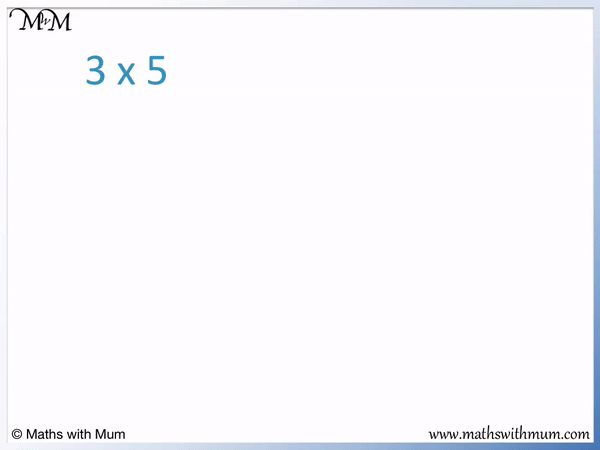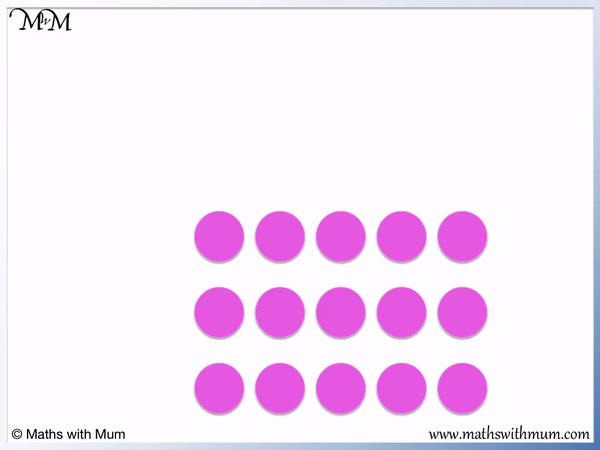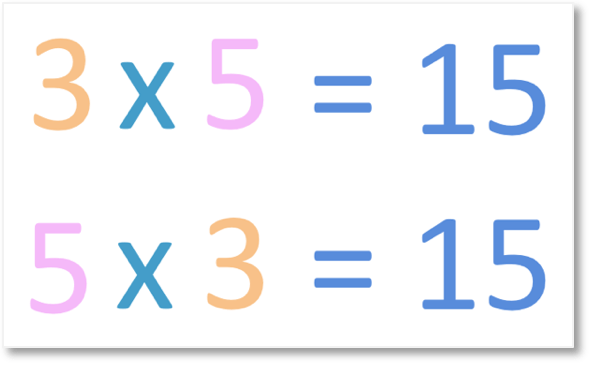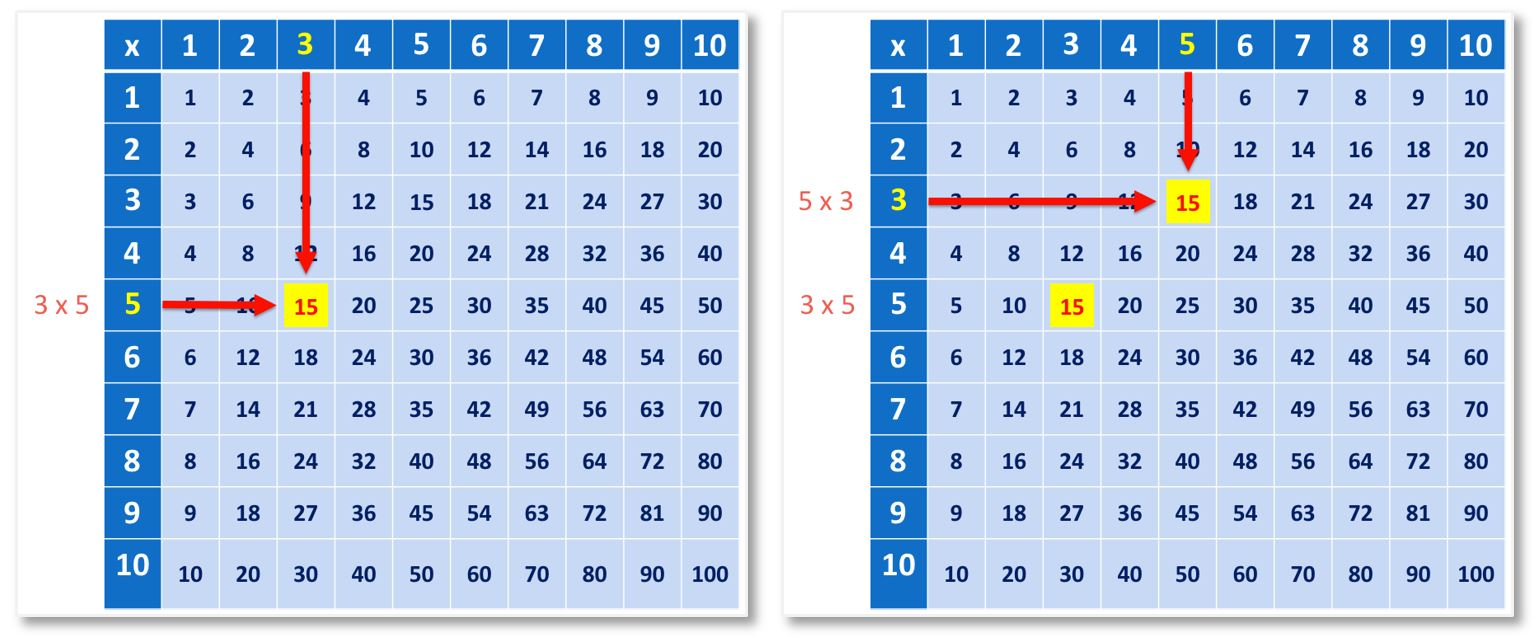# What is the Commutative Property of Multiplication?

What is the Commutative Property of Multiplication?• The commutative property of multiplication is a rule that means that it does not matter in which order numbers are multiplied.
• For example, 3 x 5 = 15 and 5 x 3 = 15. Switching the order of the 3 and the 5 did not change the answer.
• To teach the commutative property of multiplication, multiplication arrays can be used.
• In the first array, we can see 3 groups of 5.
• In the second array, we can see 5 groups of 3.
• We can see that there is still the same number of counters in each array.
The commutative property of multiplication means that changing the the order in which numbers are multiplied does not change the answer.• We can see the commutative property of multiplication on a multiplication grid.
• 8 x 5 = 40 and 5 x 8 = 40.Supporting Lessons

# The Commutative Property of Multiplication

## What is the Commutative Property of Multiplication

The commutative property of multiplication is a rule in mathematics that means that the order of multiplication does not matter. In simple terms, switching the order of the numbers being multiplied does not change the answer. The commutative property of multiplication states that a × b = b × a. For example, 3 × 5 = 15 and 5 × 3 = 15.The order of multiplication does not matter.

## How to Teach the Commutative Property of Multiplication

To teach the commutative property of multiplication, multiplication arrays can be used. A collection of counters can be arranged in an array and grouped into amounts showing each number. For example, 15 counters can be grouped into 3 groups of 5 and then 5 groups of 3 to show that the order does not matter.

Here are 15 counters.

We can make 3 lots of 5. This represents 3 × 5.We can take the same group of counters and group them as 5 lots of 3.

This represents 5 × 3.Since the total number of counters did not change, the result must be the same.

When teaching the commutative property of multiplication, use physical objects such as counters arranged in a multiplication array. Gather the counters into groups of each number to show that the total number is the same.

Alternatively, another way to teach the commutative property is to learn the times tables or use a multiplication grid and simply compare the answers to each.

If we know that 3 × 5 = 15 and that 5 × 3 = 15, we can easily compare the two multiplication sentences to discover the commutative law. The order of multiplication does not matter because the answer is the same.A multiplication grid is a grid that has numbers along the top and side. The numbers on the top of each column and side of each row are multiplied to make the numbers in each grid.

We can see that 3 × 5 = 15 and 5 × 3 = 15.A multiplication grid can be provided and then the answers compared to see that they are the same. When teaching the commutative property, you can look for pairs of common answers in the multiplication grid.

Here is an example of the commutative property, 8 × 5 = 40 and 5 × 8 = 40. The order of the multiplication does not matter.Now try our lesson on How to Find Multiples where we learn how to find the multiples of any number.